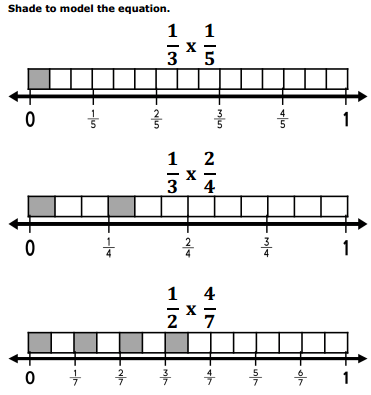# Grade 5 Parts And Wholes Worksheets

## Class 5 Maths Parts and Wholes Worksheets

1. Write as fraction:
(a)(b)(c)(d)(e)(f)2. What is the fraction of the shaded area?3. Shade the following figures with the indicated fraction.4. Write the denominator and numerator of the following fractions:5. Look at the grid, colour as directed.(a) 1/2 blue
(b) 3/8 green
(c) 2/4 red
(d) 1/8 violet

6. Solve the following:
(a) 1/2 of 18 = ……………….
(b) 2/4 of 12 = ……………….
(c) 1/3 of 27 = ……………….
(d) 3/8 of 32 = …………………
(e) 1/10 of 100 = ……………..
(f) 2/8 of 16 = …………………

7. Choose the correct answer and colour accordingly:8. Fill in the blanks:
(a) 25 paise is …………….. part of one rupee.
(b) 50 paise is …………….. part of one rupee.
(c) How many 10 paise will make one rupee? ……………..
(d) How many 20 paise will make one rupee? …………….
(e) ………………….. 25 paise will make one rupee.
(f) How many 50 paise will make one rupee? ……………..
(g) How many 5 paise will make one rupee? ………………
(h) 75 paise is ………………. part of one rupee?

9. Divide the rhombus into 4 equal parts. Shade 3 parts.
What part of fraction shaded? ……………..
What part of fraction not shaded? …………….10. Divide the rectangle into 5 equal parts. Shade 2 parts
What part of fraction shaded? ……………..
What part of fraction not shaded? ……………..11. Divide the triangle into 2 equal parts. Shade 1 part.
What part of fraction shaded? ………………..
What part of f raction not shaded? ………………12. Divide the circle into 8 equal parts. Shade 5 parts.
What part of fraction shaded? ………………..
What part of f raction not shaded? ……………13. Divide the trapezoid into 2 equal parts. Shade 1 part.
What part of fraction shaded? ……………..
What part of fraction not shaded? ……………….14. Divide the hexagon into 6 equal parts. Shade 5 parts.
What part of fraction shaded? ………………
What part of fraction not shaded? ………………15. Refer the shopping list and answer the questions that follow:

 Item Price in ₹ (per kg) Carrot 32 Potato 20 Tomato 34 Onion 42 Brinjal 38

(a) How much does 2 kg of tomato cost?
(b) How much does$\frac{1}{2}$ kg of onion cost?
(c) How much does 2$\frac{1}{2}$ kg of brinjal cost?
(d) What is the price of 1$\frac{1}{4}$ kg of carrot?
(e) Kiran bought 3$\frac{1}{2}$ kg of kg tomato. What is its cost?
(f) What is the total amount of 4 kg onion and 3 kg potato?